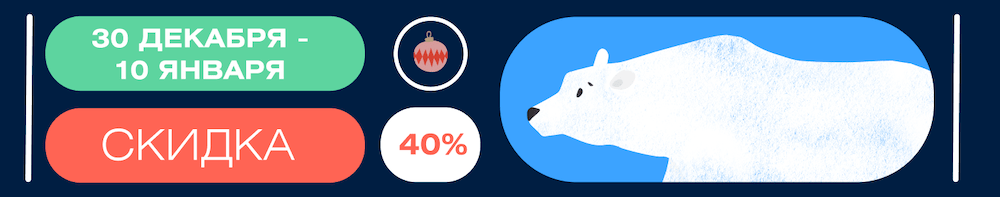Visualizing Intersections and Overlaps with Python

Exploring options for solving one of the most difficult data visualization problems

The overriding challenge in any data analysis is comparing multiple sets of something. These can be lists of IP addresses for each landing page on your website, customers who have purchased certain items from your store, multiple responses from a survey, and more.Venn diagram

In the following examples, I will use dataset of 2020 data visualization census society

I will work with the survey because there are many different types of questions; some of them are multiple choice multiple answer questions as shown below.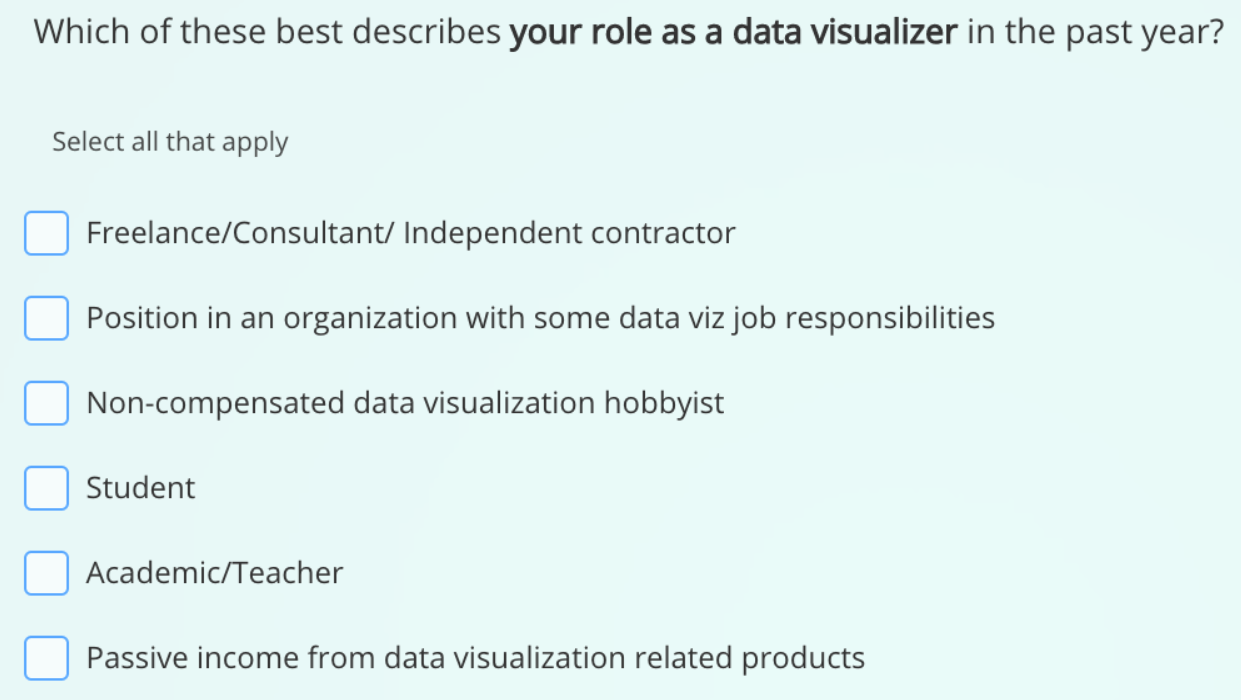A source – Datavisualizationsurvey Git

Let’s say we count each answer. In our chart, the final numbers will be greater than the total number of respondents, which can cause difficulties for the audience to understand, questions will be raised, and misunderstandings will make the audience skeptical about the data.

For example, if we had 100 respondents and three possible answers – A, B and C.

We might have something like this:
50 answers – A and B;
25 answers – A and C;bar chart

It looks confusing. Even if we explain to the audience that the respondent can choose several answers, it is difficult to understand what this diagram is.

In addition, with this visualization, we have no information about the intersection of responses. For example, it cannot be said that no one chose all three options.

Venn diagrams

Let’s start with a simple and very familiar solution – Venn diagrams. I use Matplotlib-Venn for this task.

import pandas as pd
import numpy as np
import matplotlib.pyplot as plt
from matplotlib_venn import venn3, venn3_circles
from matplotlib_venn import venn2, venn2_circles

Now let’s load the dataset and prepare the data we want to analyze.

The question we’ll test is, “Which of these best describes your role as a data visualizer over the past year?”

The answers to this question are spread across 6 columns, one for each answer. If the respondent selected an answer, the text will appear in the field. If not, the field will be empty. We will transform this data into 6 lists containing the indexes of the users who selected each answer.

nm = 'Which of these best describes your role as a data visualizer in the past year?'
d1 = df[~df[nm].isnull()].index.tolist() # independent
d2 = df[~df[nm+'_1'].isnull()].index.tolist() # organization
d3 = df[~df[nm+'_2'].isnull()].index.tolist() # hobby
d4 = df[~df[nm+'_3'].isnull()].index.tolist() # student
d5 = df[~df[nm+'_4'].isnull()].index.tolist() # teacher
d6 = df[~df[nm+'_5'].isnull()].index.tolist() # passive income

Venn diagrams are easy to understand and use.

We need to pass in sets of keys / sentences that we will analyze. If this is the intersection of two sets, use Venn2; if it’s three sets then use Venn3.

venn2([set(d1), set(d2)])
plt.show()Venn diagram

Great! With the help of Venn diagrams, we can clearly show that 201 respondents chose A and did not choose B, 974 respondents chose B and did not choose A, and 157 respondents chose A and B.

You can even customize some aspects of the schedule.

venn2([set(d1), set(d2)],
set_colors=('#3E64AF', '#3EAF5D'),
set_labels = ('FreelancenConsultantn
Independent contractor',
'Position in an organizationnwith some data
viz job responsibilities'),
alpha=0.75)
venn2_circles([set(d1), set(d2)], lw=0.7)
plt.show()venn3([set(d1), set(d2), set(d5)],
set_colors=('#3E64AF', '#3EAF5D', '#D74E3B'),
set_labels = ('FreelancenConsultantn
Independent contractor',
'Position in an organizationnwith some data
viz job responsibilities',
alpha=0.75)
venn3_circles([set(d1), set(d2), set(d5)], lw=0.7)

plt.show()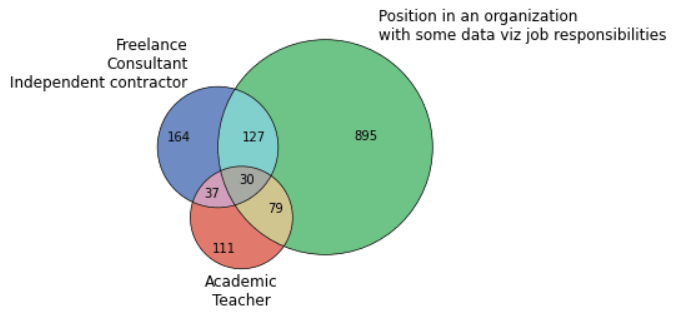This is great, but what if we wanted to display overlapping sets of more than three sets? There are a couple of possibilities here. For example, we could use multiple charts.

labels = ['FreelancenConsultantnIndependent contractor',
'Position in an organizationnwith some data viznjob responsibilities',
'Non-compensatedndata visualization hobbyist',
'Student',
'Passive income fromndata visualizationnrelated products']
c = ('#3E64AF', '#3EAF5D')
# subplot indexes
txt_indexes = [1, 7, 13, 19, 25]
title_indexes = [2, 9, 16, 23, 30]
plot_indexes = [8, 14, 20, 26, 15, 21, 27, 22, 28, 29]
# combinations of sets
title_sets = [[set(d1), set(d2)], [set(d2), set(d3)],
[set(d3), set(d4)], [set(d4), set(d5)],
[set(d5), set(d6)]]
plot_sets = [[set(d1), set(d3)], [set(d1), set(d4)],
[set(d1), set(d5)], [set(d1), set(d6)],
[set(d2), set(d4)], [set(d2), set(d5)],
[set(d2), set(d6)], [set(d3), set(d5)],
[set(d3), set(d6)], [set(d4), set(d6)]]
fig, ax = plt.subplots(1, figsize=(16,16))
# plot texts
for idx, txt_idx in enumerate(txt_indexes):
plt.subplot(6, 6, txt_idx)
plt.text(0.5,0.5,
labels[idx+1],
ha="center", va="center", color="#1F764B")
plt.axis('off')
# plot top plots (the ones with a title)
for idx, title_idx in enumerate(title_indexes):
plt.subplot(6, 6, title_idx)
venn2(title_sets[idx], set_colors=c, set_labels = (' ', ' '))
plt.title(labels[idx], fontsize=10, color="#1F4576")
# plot the rest of the diagrams
for idx, plot_idx in enumerate(plot_indexes):
plt.subplot(6, 6, plot_idx)
venn2(plot_sets[idx], set_colors=c, set_labels = (' ', ' '))
plt.savefig('venn_matrix.png')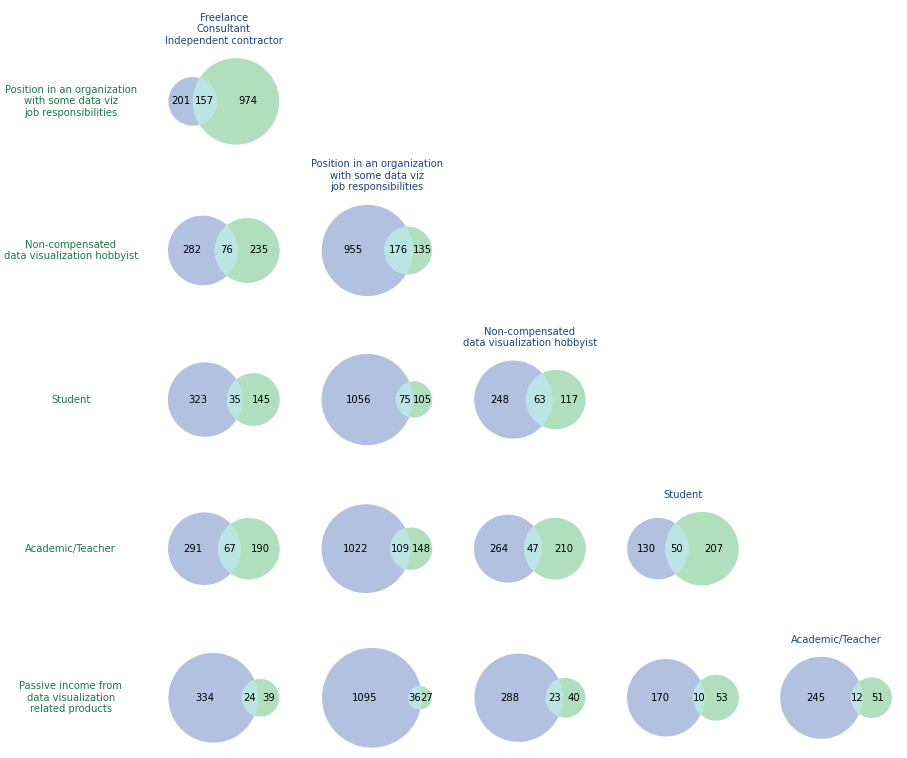Venn diagram matrix

No big deal, but that didn’t fix the problem. We cannot determine if there is someone who selected all the answers, and it is also impossible to determine the intersection of the three sets. How about a Venn diagram with four circles?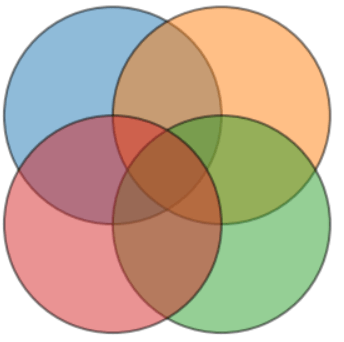This is where things start to get complicated. In the image above, there is no intersection, only blue and green. To solve this problem, we can use ellipses instead of circles.

The following two examples apply PyVenn

from venn import venn
sets = {
labels: set(d1),
labels: set(d2),
labels: set(d3),
labels: set(d4)
}
fig, ax = plt.subplots(1, figsize=(16,12))
venn(sets, ax=ax)
plt.legend(labels[:-2], ncol=6)Here it is!

But we lost size — critical information for the chart. Blue (807) is less than yellow (62), which is not very helpful in rendering. To understand which is which, we can use the legend and labels, but the table would be clearer.

There are several implementations of spatial proportional Venn diagrams that can work with more than three sets, but I could not find any in Python.

UpSet chart

But there is also another solution. UpSet charts Is a great way to display the intersection of multiple sets. They are not as intuitive to read as Venn diagrams, but they do the job. I will use UpSetPlotbut I’ll prepare the data first.

upset_df = pd.DataFrame()
col_names = ['Independent', 'Work for Org', 'Hobby', 'Student', 'Academic', 'Passive Income']
nm = 'Which of these best describes your role as a data visualizer in the past year?'
for idx, col in enumerate(df[[nm, nm+'_1', nm+'_2', nm+'_3', nm+'_4', nm+'_5']]):
temp = []
for i in df[col]:
if str(i) != 'nan':
temp.append(True)
else:
temp.append(False)
upset_df[col_names[idx]] = temp

upset_df['c'] = 1
example = upset_df.groupby(col_names).count().sort_values('c')
exampleWith the correct data positioning, we only need one method to draw our chart, and that’s it.

upsetplot.plot(example['c'], sort_by="cardinality")
plt.title('Which of these best describes your role as a data visualizer in the past year?', loc="left")
plt.show()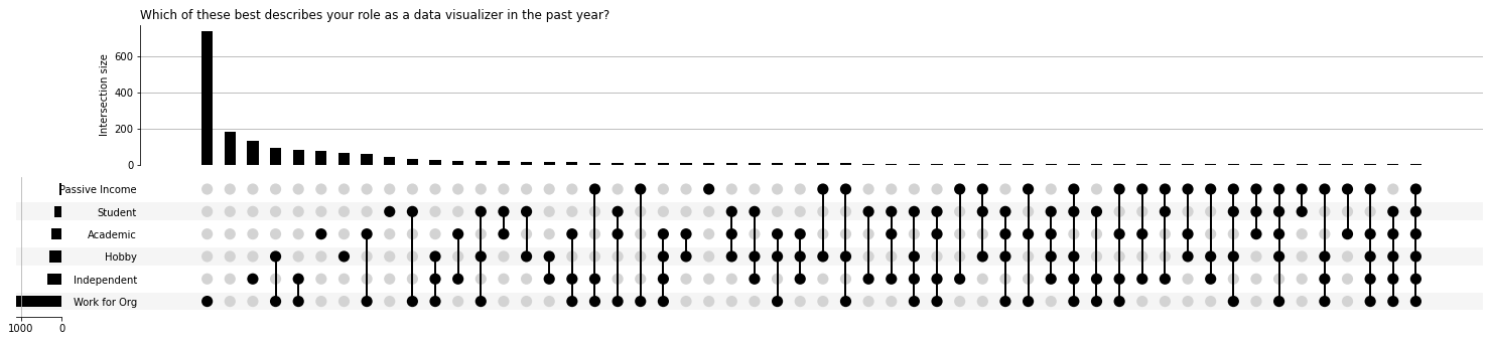UpSet chart

Awesome! At the top are columns showing how many times the combination has appeared. At the bottom is a matrix showing which combination each bar represents, and at the bottom left is a horizontal bar graph representing the total size of each set.

It’s a lot of information, but a well-organized layout makes it easy to retrieve.

Even with my badly written labels, we can easily see that most people have chosen to “work for the organization.”

The second most common answer didn’t even show up in previous Venn diagrams: the number of people who chose no answer.

In general, visualizing sets and their intersections can be a mental challenge, but we have some good options for solving it.

I prefer Venn diagrams when dealing with a small number of sets, and Upset charts when there are more than three sets. It is always helpful to explain what the visualization shows and how to read the diagrams that you present, especially in cases where the diagrams are not very friendly.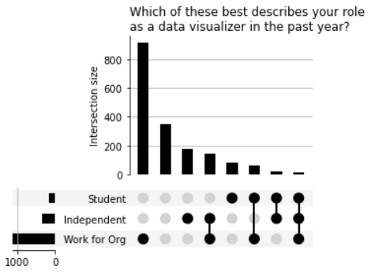Rendering of three sets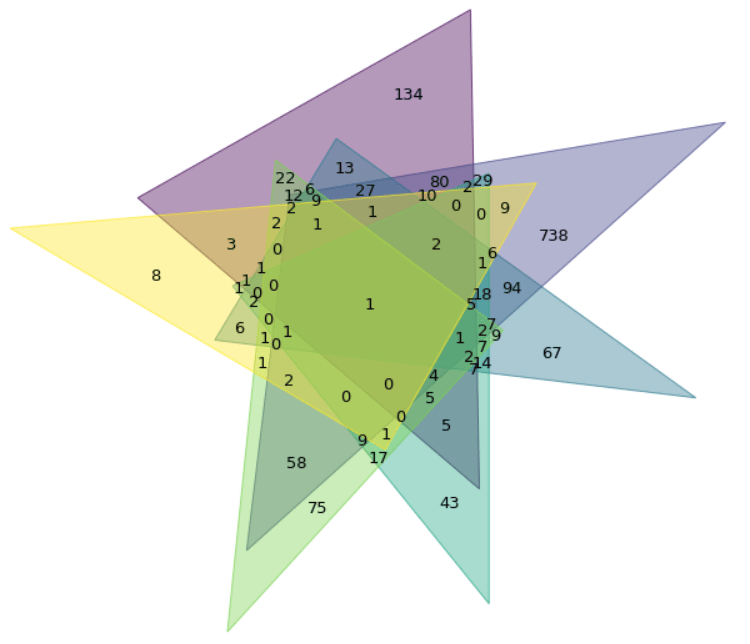Visualization of six sets